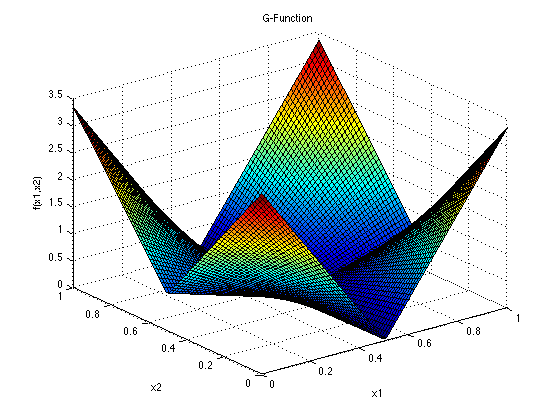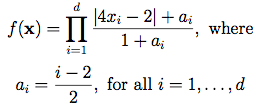## G-Function### Description:

Dimensions: d

As discussed by Marrel et al. (2009), this test function is used as an integrand for various numerical estimation methods, including sensitivity analysis methods, because it is fairly complex, and its sensitivity indices can be expressed analytically. The exact value of the integral with this function as an integrand is 1.

For each index i, a lower value of ai indicates a higher importance of the input variable xi. Above are the values of ai recommended by Crestaux et al. (2007).

### Input Domain and Distributions:

For integration, screening and sensitivity analysis, the function is integrated over the hypercube [0, 1], for all
i = 1, …, d.

For the purposes of uncertainty quantification, the independent distributions of the input random variables are, as given by Marrel et al. (2009): xi ~ Uniform[0, 1], for all i = 1, …, d.

### Modifications and Alternative Forms:

Kucherenko et al. (2011) use the values a0 = a1 = 0 and a3 = … = ad = 6.52. When used with ai = 6.52, for all i = 1, … d, they classify it as a Type B function, meaning that it has dominant low-order terms and a small effective dimension.

In many cases, for instance in Marrel et al. (2008), there is the constraint that ai ≥ 0. They conclude that:
• for ai = 0, xi is very important
• for ai = 1, xi is relatively important
• for ai = 9, xi is non-important
• for ai = 99, xi is non-significant

### References:

Crestaux, T., Martinez, J.-M., Le Maitre, O., & Lafitte, O. (2007). Polynomial chaos expansion for uncertainties quantification and sensitivity analysis [PowerPoint slides]. Retrieved from SAMO 2007 website: http://samo2007.chem.elte.hu/lectures/Crestaux.pdf.

Davis, P. J., & Rabinowitz, P. (1984). Methods of numerical integration, second ed. New York: Academic Press.

Glen, G., & Isaacs, K. (2012). Estimating Sobol sensitivity indices using correlations. Environmental Modelling & Software.

Kucherenko, S., Feil, B., Shah, N., & Mauntz, W. (2011). The identification of model effective dimensions using global sensitivity analysis. Reliability Engineering & System Safety, 96(4), 440-449.

Marrel, A., Iooss, B., Van Dorpe, F., & Volkova, E. (2008). An efficient methodology for modeling complex computer codes with Gaussian processes. Computational Statistics & Data Analysis, 52(10), 4731-4744.

Marrel, A., Iooss, B., Laurent, B., & Roustant, O. (2009). Calculations of sobol indices for the gaussian process metamodel. Reliability Engineering & System Safety, 94(3), 742-751.

Saltelli, A., Chan, K., & Scott, E. M. (Eds.). (2000). Sensitivity analysis (Vol. 134). New York: Wiley.

Saltelli, A., & Sobol', I. Y. M. (1994). Sensitivity analysis for nonlinear mathematical models: numerical experience. Matematicheskoe Modelirovanie, 7(11), 16-28.

Saltelli, A., & Sobol', I. M. (1995). About the use of rank transformation in sensitivity analysis of model output. Reliability Engineering & System Safety, 50(3), 225-239.